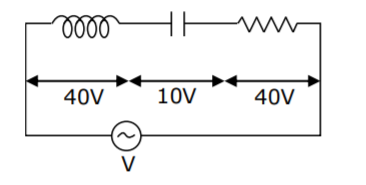An inductor of inductance L, a capacitor of capacitance C and a resistor of resistance ‘R’ are connected in series to an ac source of

An inductor of inductance L, a capacitor of capacitance C and a resistor of resistance ‘R’ are connected in series to an ac source of potential difference ‘V volts as shown in figure. Potential difference across L, C and R is 40V, 10V and 40V, respectively. The amplitude of current flowing through LCR series circuit is 10 $$\sqrt2$$ A. The impedance of the circuit is :(1) 4√2Ω (2) 5√2Ω (3) 4Ω (4) 5Ω